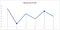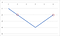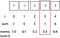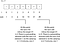# Linking Dynamic Programming (DP) challenges in LeetCode

What is DP again? Coding the answer in Swift, does that offend you? Sorry.

Prerequisites:

• None

Terminology

DP: Dynamic programming A method for solving problems by breaking them down into similar subproblems.

# LeetCode 121. Best time to buy and sell stock

## The problem

For an array of prices, the iᵗʰ element is the price of a stock on day i. How can you make the maximum profit, given that you may only make one buy and one sell action?

## The example: solution

With an input of 7,1,5,3,6,4 we take the trough (smallest buy value) from the left, and the highest sell value after this buy value. This gives us a buy value of 1, and a sell value of 6 as below. The best profit that can be achieved with this data is therefore 6–1, a maximum profit of 5.The maximum profit is 3 for this data set

## The brute force solution

We try each element as the buy element (i, but not including the last element), and then try each element after the buy element as the sell element (j).

The code looks like this:

## An (incorrect) more efficient version

My first instinct was to store the minimum buy prices, and the maximum sell price, traversing the array and changing a local min and local max to the minimum or maximum value (respectively). However, this allows us to choose the maximum before the minimum and therefore selling stock before buying it! Not the best solution!

## An more efficient version

For any given minimum we calculate the maximum profit (at any given maxium.

That is, we traverse the array and for each we update the minimum price found (so far).

We could keep the maximum profit found (so far) as an array, or could use a simple variable to store this single value.

Using this single variable for the max profit we have:

## A Dynamic Programming (DP) solution

Note: this is not actually the best way for the easy problem above, but helps with understanding the DP solutions that will follow.

The `memo` stores maximum profit from the proceeding elements. that is the previous minimum price (no matter where that occured).

Here is the algorithm:

• Set the minimum price `minPrice` to be the first element in the `prices` array. This is also stored in the memorization array `memo` in the first position `memo`.

Now traverse each remaining element `i` in `prices`, repeat the following steps:

• Where a new `minPrice` is found, use this as the `minPrice` going forwards (and if not leave it unchanged)
• Where the current price `prices[i]` is smaller than `minPrice`, reset using `minPrice = prices[i]`
• Calculate the profit using `prices[i] — minPrice` , and if it larger than `memo[i — 1]` store it in `memo[i]`. If not carry the old largest profit `memo[i-1]` forwards through `memo[i] = memo[i-1]`

Set the initial `minPrice` to be 7, and `memo` represents the maximum profit as 0 — and there is no need to traverse the first element in `prices`. so start at `i` = 1 ( which represents time point 2 on the graph since the array is zero-indexed).
Current status: `minPrice == 7, memo == [0,0,0,0,0,0]`

At time point 2 we have found a new minimum price (`prices == 1`) and record it in `minPrice` as `prices < minPrice`. We therefore carry on the previous memo value `memo == memo`.
Current status: `minPrice == 1, memo == [0,0,0,0,0,0]`

At time point 3 the price (5) is larger than `minPrice` (1) as `prices > minPrice` so `minPrice` is unchanged. The current profit is 5 -1 (4) which is more profit than the current 0, so we store 4 in the memo `memo == 4`.
Current status: `minPrice == 1, memo == [0,0,4,0,0,0]`

At time point 4 the price (3) is larger than `minPrice` (1) as `prices > minPrice` so `minPrice` is unchanged. The current profit is 3–1 (2) which is less profit than the current 4, so we continue with 4 making `memo = memo`.
Current status: `minPrice == 1, memo == [0,0,4,4,0,0]`

At time point 5 the price (6) is larger than `minPrice` (1), as `prices > minPrice` so `minPrice` is unchanged. The current profit is 6–1(5) which is more profit than the current 4 stored in `memo`, so we store the value 5 at the current point in the memo `memo = 5`.
Current status: `minPrice == 1, memo == [0,0,4,4,5,0]`

At time point 6, the price (4) is larger than `minPrice` (1), as `prices > minPrice` so `minPrice` is unchanged. The current profit is 4–1 (3) which is less profit than the current 5 stored in `memo`, so we continue with 5 at the current point in the memo `memo = 5`.
Current status: `minPrice == 1, memo == [0,0,4,4,5,5]`

The maximum profit to be gleaned from the algorithm is therefore 5 — as the largest value is memo is just that: `max(memo) == 5`

## A Dynamic Programming (DP) solution using less space

Yes! We don’t need to process the whole memo array as we are just using the largest profit at any particular point.

So we can simply use a variable to store the maximum profit so far!

You might notice that this is rather similar to a solution we developed earlier in this Medium post. Well, we now have multiple ways to get to the same solution, which is a good thing(!)

# LeetCode 525. Contiguous Array

## The problem

Given an array containing zeros and ones, find the maximum contiguous subarray containing an equal number of zeros and ones.

## The solution

To find the maximum length with an equal number of 0’s and 1’s we keep track of the proceeding number of 0’s and 1’s.

For an array, say [0,0,0,0,1,1] the maximum subarray with an equal number of 0’s and 1’s is 4 (the array being [0,0,1,1]). This is similar to the odd-even array problem that I’ve previously written about.

This time our memo will store the maximum distance between the values with an equal number of zeros and ones.

For example, if we consider each 0 to be negative, and each 1 to be positive we can create a graph (similar to those for problem 121)The sample graph for array 

We can see from the graph there are two possible points where there is an equal number of 1’s and 0’s, one of length 4 (6–2) and one of length 2 (5–3).

By extending the array to 0,0,0,0,1,1,0,0,1,1 we can see the danger — there are two possible points that have crossed “-2” and we only want to take the first one rather than the one in the middle.

To overcome this we use a memo for dynamic programming. This memo stores the “paired number” against the first index at which that has been seen.

In the example above we get the following memo output:

So we look out for the previous element in the memo that has that particular “sum” for 0’s and 1’s.

The only difference here is that 0: -1 is required to be used for the initial memo value. i.e:

var memo = [0:-1]

This is because we assume that the initial value is balanced, that is having an equal number of 0’s and 1’s (so that is why it is at the nonsensical index of -1, although this does play nicely with the zero-indexed array).

# LeetCode 325. Maximum size subarray sum equals k

## The problem

Given an array `nums` and a target value `k`, find the maximum length of a subarray that sums to `k`. If there isn’t one, return 0 instead.

## Some examples

So an array `nums` contains 1, -1, 5, -2, 3 happens to have a maximum size subarray that sums to 3 of 1, -1, 5,-2.

Similarly an array `nums` of -2, -1, 2, 1 has a maximum size subarray that sums to 1 of -1, 2.

Having seen some examples, let us move straight into the DP solution.

## A Dynamic Programming (DP) solution

We will traverse every element in the input array, using a memoization array `memo` that stores the current sum and the position of that sum.

We shall iterate along the array `nums`, and we shall call the pointer `i`. In `memo` we store the position of the sum. i.e. `memo[sum] = i`. The sum is easily calculated at any given point. `sum +=nums[i]`.

Possibility 1 — the sum is the target k

When we reach a element i, the current sum is the target (k).An implementation looking for k=3

For the example above, this gives us a length of 4. At this point, the candidate length must be the largest possible length at this point (since we move from i = 0).

No DP required!

if the current sum = k, we simply record the length (and since the array is zero-indexed this value will be i + 1)

if (sum == k) { largestLength = i+1 }

Possibility 2 — look for a proceeding subarrayAn example array with two subarrays.

Before this example, we can actually see something interesting.

By keeping track of the sum we have the opportunity for any element i within the array, if there is a previous sum equal to k — sum.

Visually:

The general case there is only a candidate max subarray is a previous element has a sum equal to sum -k.

And of course this needs to be repeated for every possible value i (and we compare each to see if it is the longest such subarray).

We can calculate the length of the subarray by storing the value i when we store the sum. The length will be i — stored value[sum — k].

In our code this will be (if and only if we have already stored the location of sum-k in the DP memo array)

largestLength = max(largestLength, i — memo[sum-k]!)

in any case we will store the sum in the dictionary

memo[sum] = i

Giving us the following Swift solution:

# LeetCode 1124. Longest performing interval

## The problem

Given an array of hours, find the longest interval where the number of tiring days is strictly larger than the number of non-tiring days.

## In-depth problem

A tiring day is such if and only if the number of hours worked is strictly greater than 8. A non-tiring day is a day such that the number of hours worked is less than 8.

We need to calculate the longest subarray where in the subarray the number of tiringDays is strictly larger than the number of nonTiringDays (tiringDays > nonTiringDays). To monitor this, we maintain a variable `countAboveZeroTiringDays`, which monitors whether we are “above the line” of tiring data and, if so, how much.

## The solution

Like the previous challenges, we will use a `memo`. This `memo` stores the number of tiring days, and the index against that so we can store the longest well performing interval — that will be the largest run stored in the memo.

This is much like the LeetCode 325 solution (shown above within this article)

We traverse the entire array `hours` , while maintaining a `maxLength` that persists across the days we traverse. Ultimately this `maxLength` is the length of the subarray that will be returned by the function.

We maintain a count above zero tiring days — and increment it if the current hour is larger than 8, and decrement if it is less than 8 (or equal).

if our current count is positive, then we are on a valid run (for index =0) so the current maxLength is set as i + 1

If not we are on a run where the current countAboveZeroTiringDays is negative (and we are done for this number).

We want a positive score so we are NOT in a trough is there is a memo with such that memo[countAboveZeroTiringDays -1]. If we are NOT in a trough then there MUST be a run of days, and if that run of days is longer than the one currently stored, then we have a new maximum length.

We continue to store the first occurrence of any negative countAboveZeroTiringDays.

This gives the solution of 3. This is because the distance between element 3 (16) and element 1 (10) is 3 (inclusive) — given in the algorithm by `i — memo[countAboveZeroTiringDays — 1]`. That is when we reach `i = 3`, the longest distance to any element that was -1 in the memo (which would be the longest away) is three since the last element at -1 was 0.

Phew!

# Conclusion

The truth is many problems on LeetCode are linked, and knowing how to complete some problems will help you work out solutions to other problems.

I hope this article gives you some indication about how to go about using Dynamic Programming and Memoization in Swift.

Good luck!

Any questions? You can get in touch with me in a Twitter rant. HERE

stevecurtis.me